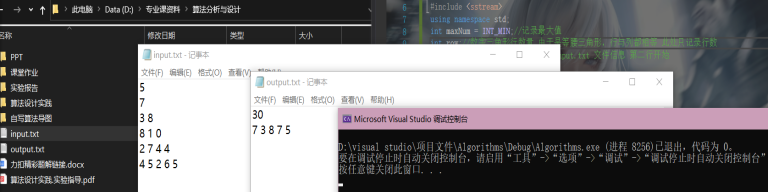# 动态规划求解数字三角形问题

8 篇文章 0 订阅

input.txt
5
7
3 8
8 1 0
2 7 4 4
4 5 2 6 5

output.txt
30
7 3 8 7 5

## 如何求解？

• 需要求的是三角形中最大路径和，一提到最值问题，动态规划对于此类问题是比较拿手的，本题使用了动态规划去求解。
• 确定dp数组含义； dp[i][j]定义为在三角形第i行第j列位置时最大的路径和。（至于为什么这么去定义，笔者并无较好建议，是通过大量做题的经验得来的）。
• 确定遍历顺序；首先我想到了从三角形的上顶点往下遍历，但在后续填充dp数组时则需要注意边界处理(最左端以及最右端都需要进行条件判断并给予相应处理)，整体将显得比较繁杂。因而换了一种思路：从三角形的底边开始网上遍历，这种方式实现起来更加简单（除去了边界判断。）。综上，最后的遍历顺序为行方向上为自下而上，列方向上从左至右；
• 确定递推公式; 根据遍历顺序，dp数组的每一行都是下一行得到（除去最后一行），例如第3行的数据是通过第4行得到的。
而每个位置只能通过正下方或者右下方到达。为了得到最大路径和：
• dp[i][j] = max(dp[i + 1][j], dp[i + 1][j + 1]) + 当前位置的数值
• 初始化dp数组; 递推公式中每一行都用到了下一行的结果，因此必须考虑到最后一行如何填充。这里有两种方式:
①创建dp数组时多出三角形一行，并且将最后一行初始化为0，这样遍历填充dp数组时可以保证不会越界以及结果的正确性。
②dp数组的最后一行的数值肯定与三角形是相同的。因此直接对dp底端赋值即可。

//只能选择正下方或者右下方作为直接后继行走
void searchPath() {
//    inf数组: 存放数字三角形各行数据
vector<vector<int>> dp = inf;//创建 row 行 row 列的二维数组

for (int i = row - 2; i >= 0; i--) {//倒数第二行开始遍历
for (int j = 0; j <= i; j++) {
dp[i][j] = max(dp[i + 1][j], dp[i + 1][j + 1]) + inf[i][j];
}
}
maxNum = dp;
backTrace(dp);
}


### 文件读写

//读取文件
stringstream ss;
string temp;//保存文件信息 ---> 信息中转站
getline(fin, temp);
ss << temp;
ss >> row;//读取第一行作为数字三角形的总行数
int i = 0;
while (getline(fin, temp)) {
vector<int> vec;
ss.clear();//每次读取之前清空以下 ss 原来的数据
ss << temp;
string s = "";
while (ss >> s) {
stringstream sS;
sS << s;
sS >> i;
vec.push_back(i);
}
inf.push_back(vec);
}
}


## 完整代码

#include <iostream>
#include <fstream>
#include <string>
#include <vector>
#include <algorithm>
#include <sstream>
using namespace std;
int maxNum = INT_MIN;//记录最大值
int row;//数字三角形行数量 由于是等腰三角形，行与列都相等 此处只记录行数
vector<vector<int>> inf;//保存 input.txt 文件信息 第二行开始
vector<int> Path;
void searchPath();//搜寻路径
void writeFile(string writefile);//写文件
void backTrace(vector<vector<int>>& dp);//回溯寻找最优路径

//读取文件
stringstream ss;
string temp;//保存文件信息 ---> 信息中转站
getline(fin, temp);
ss << temp;
ss >> row;//读取第一行作为数字三角形的总行数
int i = 0;
while (getline(fin, temp)) {
vector<int> vec;
ss.clear();//每次读取之前清空以下 ss 原来的数据
ss << temp;
string s = "";
while (ss >> s) {
stringstream sS;
sS << s;
sS >> i;
vec.push_back(i);
}
inf.push_back(vec);
}
}
//只能选择正下方或者右下方作为直接后继行走
void searchPath() {
vector<vector<int>> dp = inf;//创建 row 行 row 列的二维数组

for (int i = row - 2; i >= 0; i--) {//倒数第二行开始遍历
for (int j = 0; j <= i; j++) {
dp[i][j] = max(dp[i + 1][j], dp[i + 1][j + 1]) + inf[i][j];
}
}
maxNum = dp;
backTrace(dp);
}
//回溯寻找最优路径
void backTrace(vector<vector<int>>& dp) {
int rowIndex = 0, colIndex = 0;
while (rowIndex < row - 1) {//最多遍历到倒数第二行
Path.push_back(inf[rowIndex][colIndex]);
int tmp = dp[rowIndex][colIndex] - inf[rowIndex][colIndex];
if (tmp == dp[rowIndex + 1][colIndex + 1])
colIndex++;
rowIndex++;
}
//最后一行单独处理
Path.push_back(inf[rowIndex][colIndex]);
}
//写入文件
void writeFile(string writefile) {
ofstream desFile(writefile);
desFile << maxNum << "\n";//最终结果写入 output.txt
for (int i = 0; i < row; i++) {
desFile << Path[i] << " ";
}
desFile.close();
}

int main() {
//读取文件路径
//写入文件路径
string writefile = (char*)"D:\\专业课资料\\算法分析与设计\\output.txt";
searchPath();
writeFile(writefile);
return 0;
}


## 运行效果12-19
01-26708007-041289
04-16332
05-291万+
04-21213
05-03517
08-053384
05-28205
02-19519
02-231196
03-31436
09-1789
03-182744
10-28201
04-143万+
12-22
11-182398
07-09777
11-041万+点击重新获取扫码支付余额充值